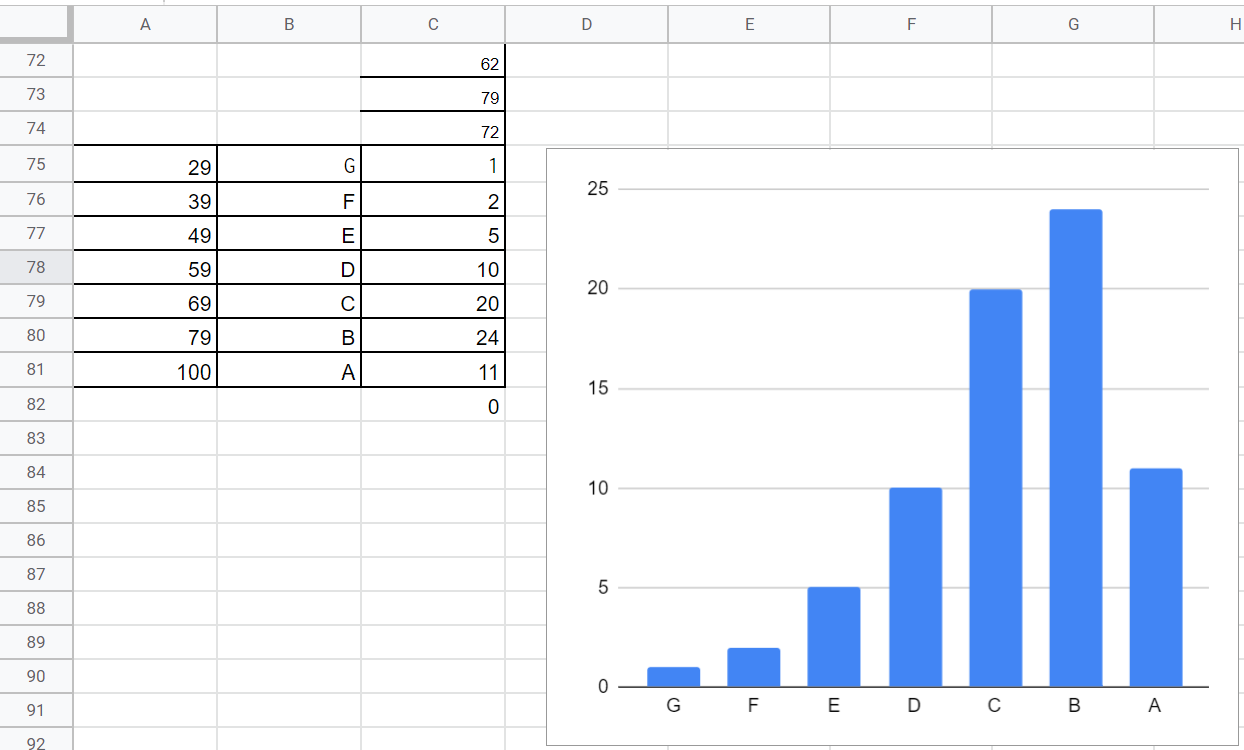# Data Analysis - Frequency

Here’s a useful trick for doing a frequency analysis in Google Sheets or Excel.

If you have a column of marks, you can use the FREQUENCY function and then draw a graph to get something that looks like this:In this example, the marks are in column C, going from row 1 to row 74.

1. In column A from starting in row 75, put in the grade boundaries: 29, 39, etc.
2. In column B from starting in row 75, put in the grade labels: G, F, E, etc.
3. In cell C75 type in this formula: =FREQUENCY(C1:C74,A75:A81). As you can see, the first range is the column of marks, the second range is the list of grade boundaries.
4. To get the graph, select cells B75 to C81 and click on the Insert Chart button. You might need to change the chart type to column.

BONUS TIP: if you want to get the frequencies for a lot of columns, you can introduce \$ into your formula as follows: =FREQUENCY(C1:C74,\$A75:\$A81) and then use autofill from C75 to D75 etc. The \$ signs keep the autofill fixed on column A. This is known as an absolute reference (as opposed to relative which changes as you autofill).